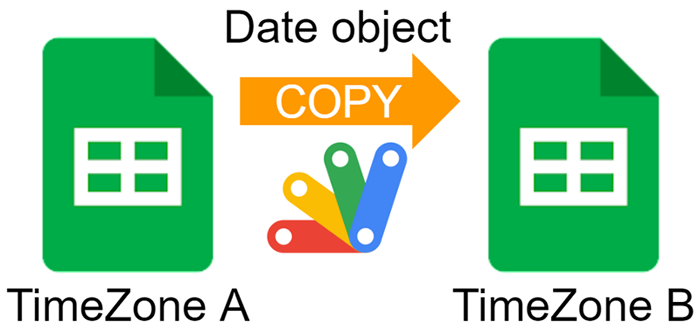GistsThis is a sample script for copying the date object between Google Spreadsheets with the different time zones using Google Apps Script.

One day, you might have a situation in which it is required to copy the date object between Google Spreadsheets with the different time zones using Google Apps Script. In this post, I would like to introduce the sample scripts for achieving this.

## Sample situation

Here, the sample situation is declared. In order to test the below scripts, please create 2 Google Spreadsheets.

1. Title: “Spreadsheet A”, timeZone “Asia/Tokyo”, As a sample date object, please put a value of “2023/01/01 12:00:00” to a cell “A1” of the 1st sheet tab.

2. Title: “Spreadsheet B”, timeZone “America/Los_Angeles” (Pacific time)

The time difference between “Asia/Tokyo” and “America/Los_Angeles” is 17 hours.

This report supposes the situation that the value of cell “A1” 2023/01/01 12:00:00 from “Spreadsheet A” to “Spreadsheet B”.

## Sample script 1

In this script, the date object of 2023/01/01 12:00:00 is copied by changing the timezone. So, 2023/01/01 12:00:00 is copied as 2022/12/31 19:00:00 by considering the time difference.

function sample1() {

);
const range = ss1.getSheets().getRange("A1");
const numberFormat = range.getNumberFormat();
const dateObject = range.getValue();
ss2
.getSheets()
.getRange("!A1")
.setNumberFormat(numberFormat)
.setValue(dateObject);
}


When this script is run, a value of 2023/01/01 12:00:00 of “A1” of the 1st tab in Spreadsheet A is copied to “A1” of the 1st tab in Spreadsheet B. When the value of 2023/01/01 12:00:00 is copied from Spreadsheet A to Spreadsheet B, the value on Spreadsheet B becomes 2022/12/31 19:00:00, because of the time difference of 17 hours between “Asia/Tokyo” and “America/Los_Angeles”.

If you want to copy the date object between Spreadsheet A and Spreadsheet B by considering the time difference, this script can be used. But, if you want to copy the date object between Spreadsheet A and Spreadsheet B with ignoring the time difference, for example, when you want to copy a date object 2023/01/01 12:00:00 between Spreadsheet A and Spreadsheet B as the same value, this script might not be suitable. In that case, the following sample scripts might be able to be used.

## Sample script 2

If the number format of the date object is the number format that can automatically detect the date object from the string type, the following script can be used.

In this script, the date object of 2023/01/01 12:00:00 is copied without considering the time difference. So, 2023/01/01 12:00:00 is copied as 2023/01/01 12:00:00.

function sample2() {

);
const range = ss1.getSheets().getRange("A1");
const numberFormat = range.getNumberFormat();
const stringValue = range.getDisplayValue();
ss2
.getSheets()
.getRange("!A1")
.setNumberFormat(numberFormat)
.setValue(stringValue);
}


In this script, the cell value of 2023/01/01 12:00:00 is retrieved as the string value with getDisplayValue(), and 2023/01/01 12:00:00 of string value is put into “A1” of 1st tab in Spreadsheet B. In the case of 2023/01/01 12:00:00 of the string value, when this is put into the cell, it is automatically converted to the date object.

However, there are cases in which the number format, which cannot be automatically converted to the date object, is used. In that case, unfortunately, this method cannot be used. So, I would like to introduce the following scripts.

## Sample script 3

In this sample, the goal is achieved by changing the spreadsheet timezone.

In this script, the date object of 2023/01/01 12:00:00 is copied without considering the time difference. So, 2023/01/01 12:00:00 is copied as 2023/01/01 12:00:00.

function sample3() {

);
const range = ss1.getSheets().getRange("A1");
const numberFormat = range.getNumberFormat();
const dateObject = range.getValue();

ss2
.getSheets()
.getRange("!A1")
.setNumberFormat(numberFormat)
.setValue(dateObject);
}


In this script, the cell value of 2023/01/01 12:00:00 is retrieved as the date object, and the timezone is changed to the same with Spreadsheet A, and 2023/01/01 12:00:00 of the date object is put into “A1” of 1st tab in Spreadsheet B. Then, the timezone of Spreadsheet B is changed to the original timezone. By this flow, 2023/01/01 12:00:00 is put into “A1” of the 1st tab in Spreadsheet B.

## Sample script 4

In this sample, the goal is achieved by converting the date object to the serial number reflecting the time difference.

In this script, the date object of 2023/01/01 12:00:00 is copied without considering the time difference. So, 2023/01/01 12:00:00 is copied as 2023/01/01 12:00:00.

function sample4() {

);
const range = ss1.getSheets().getRange("A1");
const numberFormat = range.getNumberFormat();
const dateObject = range.getValue();

const unixTimeWithOffset =
dateObject.getTime() - 1000 * 60 * dateObject.getTimezoneOffset();
const serialNumber = unixTimeWithOffset / 1000 / 86400 + 25569; // Ref: https://stackoverflow.com/a/6154953
ss2
.getSheets()
.getRange("!A1")
.setNumberFormat(numberFormat)
.setValue(serialNumber);
}


In this script, the cell value of 2023/01/01 12:00:00 is retrieved as the date object, and the date object is converted to the serial number with reflecting the time difference. Then, the serial number is put into “A1” of the 1st tab in Spreadsheet B. By this flow, 2023/01/01 12:00:00 is put into “A1” of the 1st tab in Spreadsheet B.

## Sample script 5

In this sample, the goal is achieved by converting the timezone of the date object using Utilities.parseDate.

In this script, the date object of 2023/01/01 12:00:00 is copied without considering the time difference. So, 2023/01/01 12:00:00 is copied as 2023/01/01 12:00:00.

function sample5() {

);
const range = ss1.getSheets().getRange("A1");
const numberFormat = range.getNumberFormat();
const dateObject = Utilities.parseDate(
range.getDisplayValue(),
range.getNumberFormat().replace(/"/g, "")
);
ss2
.getSheets()
.getRange("!A1")
.setNumberFormat(numberFormat)
.setValue(dateObject);
}


In this script, the cell value of 2023/01/01 12:00:00 is retrieved as the date object, and the date object is converted by giving the timezone of Spreadsheet B. And then, the converted date object is put into “A1” of the 1st tab in Spreadsheet B. By this flow, 2023/01/01 12:00:00 is put into “A1” of the 1st tab in Spreadsheet B.